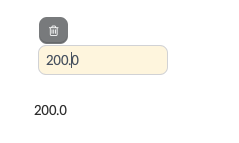# Variables

There are several tags you can use to create new variables.

## Assign

Assign tag is used to assign a value to a variable.
When you assign a value to a variable, you will create a string.

``````{% assign profit = 100 %}

{{ profit }}
``````
Output
100

### Running totals

You can use variables to setup a running total of your numeric values. To do so, set your total variable to 0 at the top to initialise it, and then update the total variable after each relevant value.

``````{% assign revenue = 130 %}
{% assign cost = 50 %}
{% assign running_total = 0 %}

{% assign running_total = running_total+revenue %}
{{ revenue }}
{% assign running_total = running_total+cost %}
{{ cost }}
{{ running_total }}
``````
Output
130
50
180

## Capture

With this function you capture the string inside of the opening and closing tags and assigns it to a variable. Variables that you create using capture are stored as strings.

``````{% assign profit = 100 %}
{% capture profit_sentence %}
Your profit is {{ profit }}.
{% endcapture %}

{{ profit_sentence }}
``````
Output

### Dynamic variables

With a combination of assign and capture you can also create something that we call a dynamic variable.
You refer to the value of a dynamic variable by using square brackets.

``````{% assign category = "equity" %}
{% capture category_total %}{{ category }}_total{% endcapture %}
{% assign [category_total] = 1000000 %}

{% comment %}Print the variable without square brackets = output of the capture{% endcomment %}
{{ category_total }}

{% comment %}Print the variable with square brackets = output of the assign{% endcomment %}
{{ [category_total] }}
``````
Output
equity_total
1000000

This is especially useful when you're working with a collection and want to create unique variables for every iteration.

## Register

❗️

Variables vs registers

We recommend using variables to calculate running totals instead of registers. The information below is still here in case you're dealing with legacy code.

Registers are used to store numbers and / or result of a calculation. This allows you to print the value or start some further calculations with the register.

Registers are local variables with some unique characteristics. Registers are created by using a dollar sign \$ with a number from 0 to 39.

### Register example

📘

Let's assume that revenue is 200.
Let's assume that cost is -100.

We want to add up two values, revenue and cost. Therefore, we store both values in 1 register (\$0). By printing \$0, we automatically see the value the register contains.

``````{% \$0+ revenue %}
{% \$0+ cost %}

{{ \$0 }}
``````
Output
100.0

It is possible to show the variables that were added by putting an equal sign.

``````Revenue: {% =\$0+ revenue %}
Cost: {% =\$0+ cost %}

Profit: {{ \$0 }}
``````
Output
Revenue: 200.00
Cost: -100.00

Profit: 100.0

### Registers with input

Register can be used in combination with an input tag.

``````{% \$0+input custom.asset.value %}

{{ \$0 }}
``````
OutputWhat’s Next